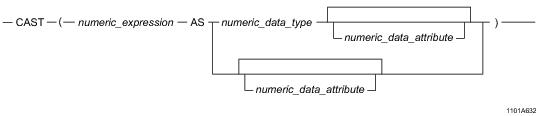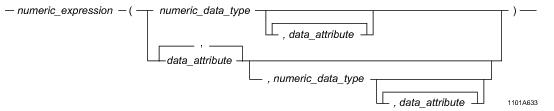# 15.00 - Numeric-to-Numeric Conversion - Teradata Database

## Teradata Database SQL Functions, Operators, Expressions, and Predicates

Product
Release Number
15.00
Content Type
Programming Reference
Publication ID
B035-1145-015K
Language
English (United States)

## Numeric-to-Numeric Conversion

### Purpose

Converts a numeric expression defined with one data type to a different numeric data type.

### CAST Syntaxwhere:

 Syntax element … Specifies … numeric_expression an expression or existing field having a numeric data type. numeric_data_type the optional numeric data type to which numeric_expression is to be converted. numeric_data_attribute one of the following optional data attributes: FORMAT NAMED TITLE

### ANSI Compliance

This is ANSI SQL:2011 compliant.

As an extension to ANSI SQL, CAST permits data attributes such as the FORMAT phrase that enables an alternative format for numeric_expression.where:

 Syntax element … Specifies … numeric_expression an expression or existing field having a numeric data type. numeric_data_type the optional numeric data type to which numeric_expression is to be converted. data_attribute one of the following optional data attributes: FORMAT NAMED TITLE

### ANSI Compliance

This is a Teradata extension to the ANSI SQL:2011 standard.

### Implicit Numeric-to-Numeric Conversion

Numeric items are converted to the same numeric type before any arithmetic or comparison operation is performed. The result returned is of this same underlying type.

For example, before an INTEGER value is added to a FLOAT value, the INTEGER value is converted to FLOAT, the data type of the result.

For details on implicit type conversions for binary arithmetic expressions, see “Binary Arithmetic Result Data Types” on page 110.

For details on implicit type conversions for comparison operations, see “Implicit Type Conversion of Comparison Operands” on page 500.

### Conversion to FLOAT/REAL/DOUBLE PRECISION

Because floating point numbers are not exact values, conversion of DECIMAL and integer values to FLOAT values might result in a loss of precision or produce a number that cannot be represented exactly. For example, a value like 0.1, when cast to FLOAT, no longer exactly equals to 0.1.

### Truncation and Rounding During Conversion

Conversion of DECIMAL/NUMERIC to BIGINT, INTEGER, BYTEINT, or SMALLINT truncates any decimal portion. Conversion to DECIMAL produces a rounded result. If a range violation occurs, the operation may fail.

Conversion to FLOAT/REAL/DOUBLE PRECISION rounds to the nearest value available. Neither decimal fractions nor numbers greater than 9,007,199,254,740,992 can be guaranteed to be represented exactly, so the nearest representable value is chosen. If there are two representable values that qualify as the nearest value, then the representation with a '0' in the least significant bit is chosen. For example, 0.1, when stored in a FLOAT column, is rounded to a value slightly higher: 0.1000000000000000055511151231257827021181583404541015625.

For details on rounding, see “Decimal/Numeric Data Types" in SQL Data Types and Literals.

Some examples of numeric conversions are:

 Value Converted To Result 20000.99 INTEGER 20000 20000.99 DECIMAL(6,1) 20001.0 20000.99 DECIMAL(4, 1) error 200000 SMALLINT error

### Using CAST in Applications With DECIMAL Type Size Restrictions

Some applications require DECIMAL types to have 15 digits or less.

Applications with this requirement may need to access DECIMAL columns that have more than 15 digits or use expressions that may produce DECIMAL results with more than 15 digits. To help with DECIMAL type size requirements, you can use CAST to convert DECIMAL types to a size of 15 or fewer digits.

For example, consider the following expression where A, B, and C are columns defined as DECIMAL(8,2):

`   SELECT (A*B)/C FROM table1;`

The resulting value may be less than 15 digits, but A*B could be up to 18.

To ensure a result of less than 16 digits, use CAST:

`   SELECT CAST ((A*B)/C AS DECIMAL(15,2)) FROM table1;`

### Using CAST To Avoid Numeric Overflow

Because of the way the Teradata SQL compiler works, it is essential that you CAST the arguments of your expressions whenever large values are expected.

For example, suppose f1 is defined as DECIMAL(14,2) and you are going to multiply by an integer or get SUM(f1).

In this case, the following operations:

`   CAST(f1 AS DECIMAL(18,2))*100`

or

`   SUM(CAST(f1 AS DECIMAL(18,2)))`

are proper techniques for ensuring correct answer sets.

On the other hand, if you were to cast the results of the expressions, such as the following:

`   CAST(f1*100 AS DECIMAL(18,2))`

or

`   CAST(SUM(f1) AS DECIMAL(18,2)`

then you will likely experience overflow during the computations (and before the CAST is made)—not the desired result.

### Example

This example casts the numeric integer expression named IntegerField to DECIMAL(7,2).

`   CAST (IntegerField AS DECIMAL (7,2))`

### Example

Although the FORMAT phrase cannot be used to change the underlying data type defined for a column, the phrase may be used to change the display for a numeric value.

For example, if the field values for columns Wholesale and Retail, both defined as DECIMAL(7,2), are 12467.75 and 21500.50, respectively, the result of the expression:

`   CAST (Wholesale - Retail AS FORMAT '-99999')`

is:

`   -09033`

A FORMAT phrase does not affect data that is returned to the client system in Record Mode (client system internal format).

In the previous example, the value returned to the client system is still in packed decimal format (for example, -9032.75).

The use of FORMAT in CAST is a Teradata extension to the ANSI standard.

### Related Topics

For details on data types and data attributes, see SQL Data Types and Literals.